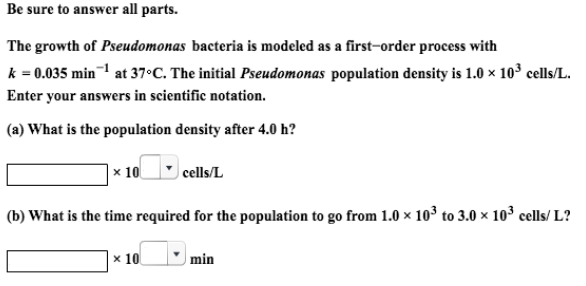# Problem: Be sure to answer all parts.The growth of Pseudomonas bacteria is modeled as a first-order process with k = 0.035 min -1 at 37°C. The initial Pseudomonas population density is 1.0 x 103 cells/L. Enter your answers in scientific notation. (a) What is the population density- after 4.0 h? (b) What is the time required for the population to go from 1.0 x 10 3 to 3.0 x 103 cells/L?

###### FREE Expert Solution
99% (48 ratings)###### Problem Details

Be sure to answer all parts.

The growth of Pseudomonas bacteria is modeled as a first-order process with k = 0.035 min -1 at 37°C. The initial Pseudomonas population density is 1.0 x 103 cells/L.

(a) What is the population density- after 4.0 h?

(b) What is the time required for the population to go from 1.0 x 10 3 to 3.0 x 103 cells/L?